Superheavy elements in the $r$-processLA-UR-19-XXXXX

Matthew Mumpower

SuperHeavy Elements
Hakone, Japan

Monday Dec. 2$^{nd}$ 2019Center for Theoretical
ASTROPHYSICS

Understanding of the formation of the elements

One of the grand challenges of our time

The theoretical modeling of astrophysical environments

Multi-messenger observations (gravitational waves, EM waves, etc.)

Nuclear theory predictions for exotic nuclei

Precision experiments to constrain nuclear theory

Data and observations are limited

We must be clever when deciphering what is going on with nucleosynthesis...What is the $r$-process?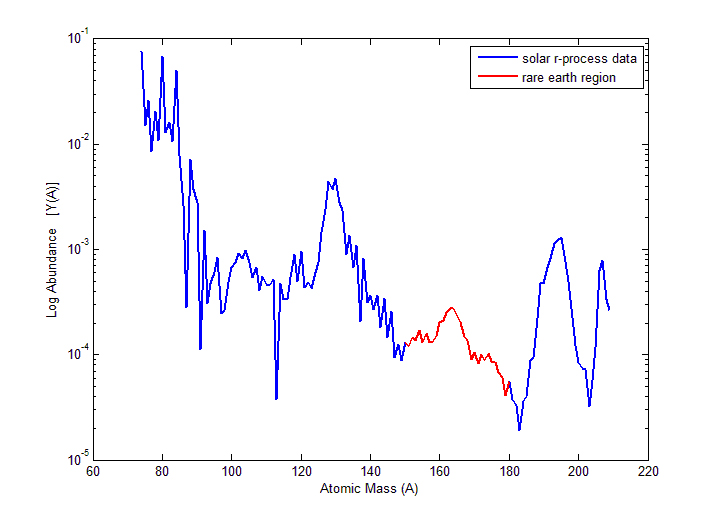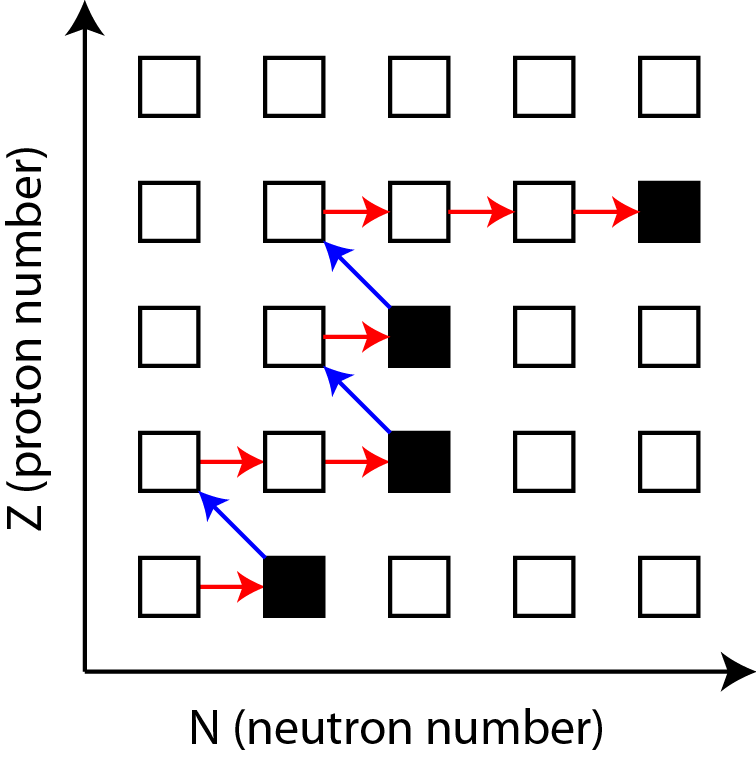The rapid neutron capture process is a cosmic factory for the production of heavy elements

Neutron captures are initially much faster than $\beta$-decays

Astrophysical environment must produce a lot of free neutrons

How far in proton number can this process proceed?...

Aprahamian et al. (2018) • Horowitz et al. J Phys G 083001 (2019) • Cowan et al. (2019)

Where can the $r$-process occur?

One possibility is in (rare?) supernovae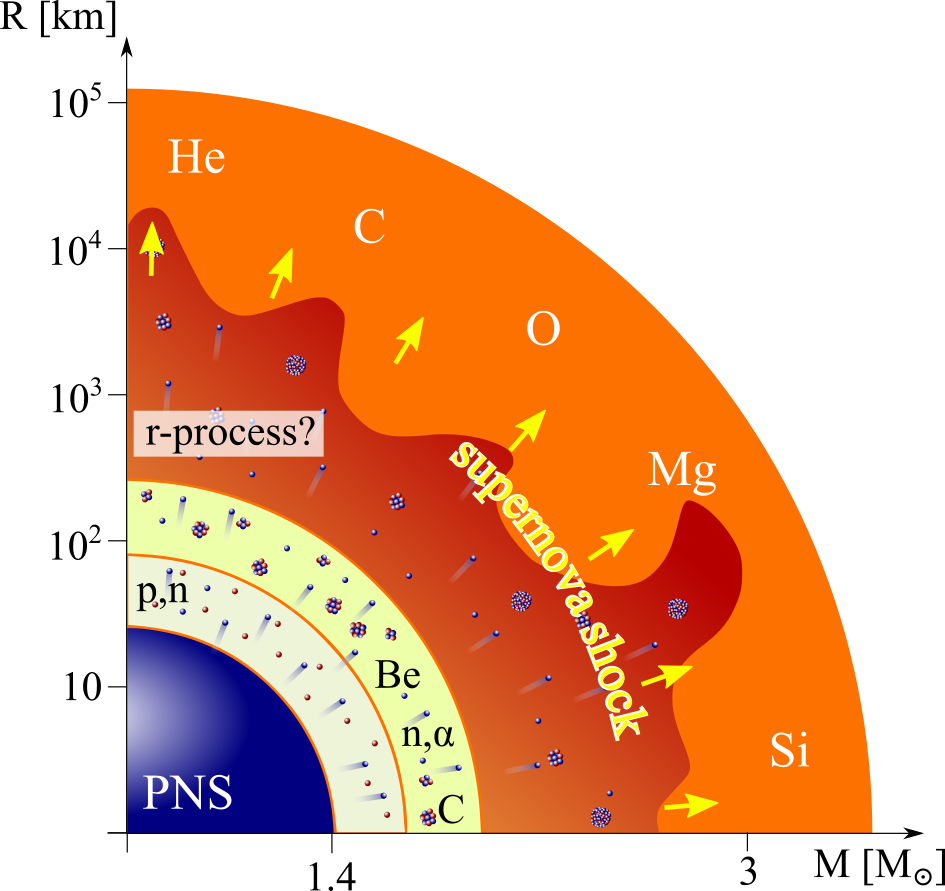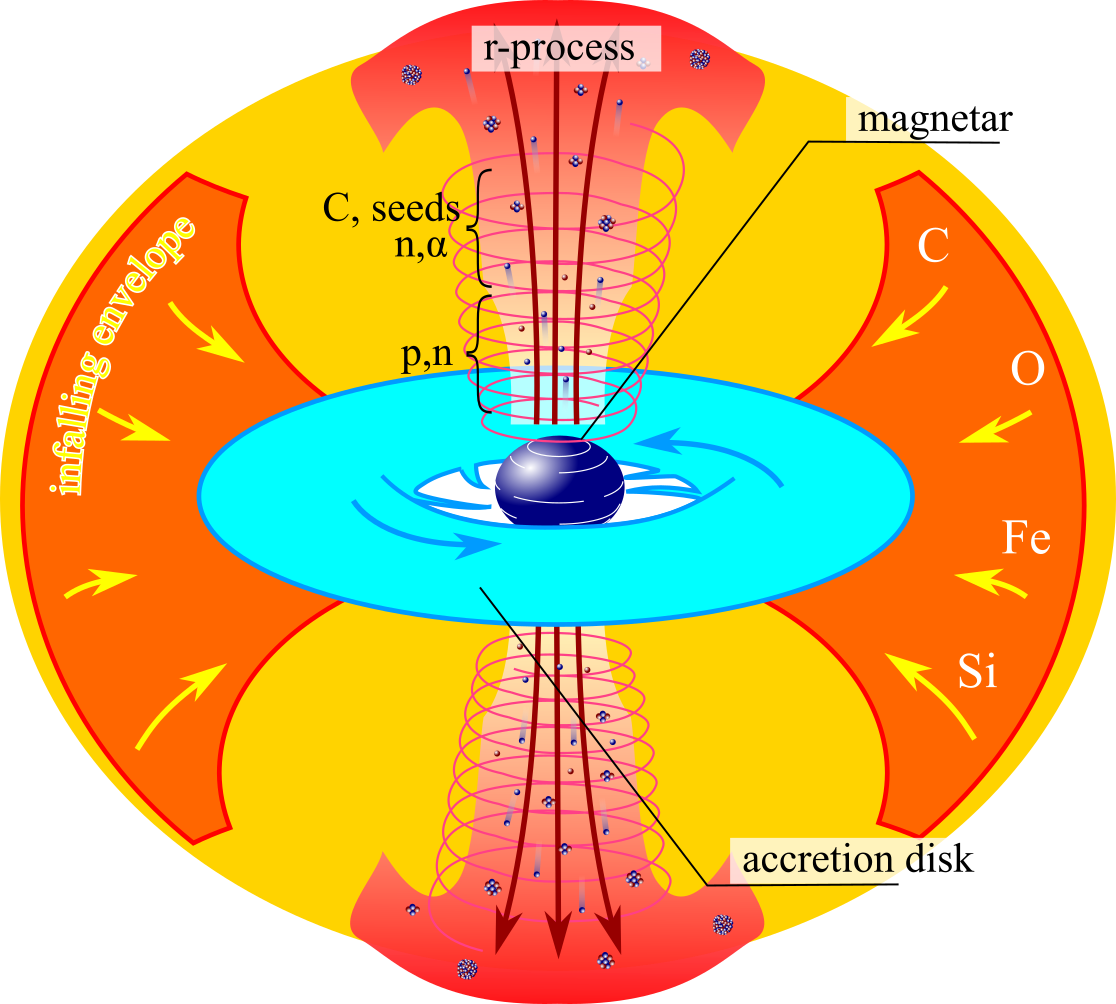For standard supernovae (left) neutrino physics still needs to be well understood

Jets in magnetorotational driven supernovae (right) may also provide the necessary conditions

Another option is the disk winds of collapsars - black hole forms after core collapse of a rapidly rotating star

Meyer ApJ (1989) • MacFadyen & Woosley ApJ (1999) • Figure from Mumpower et al. in prep. (2019)

Where can the $r$-process occur?

Another possibility is in compact object mergers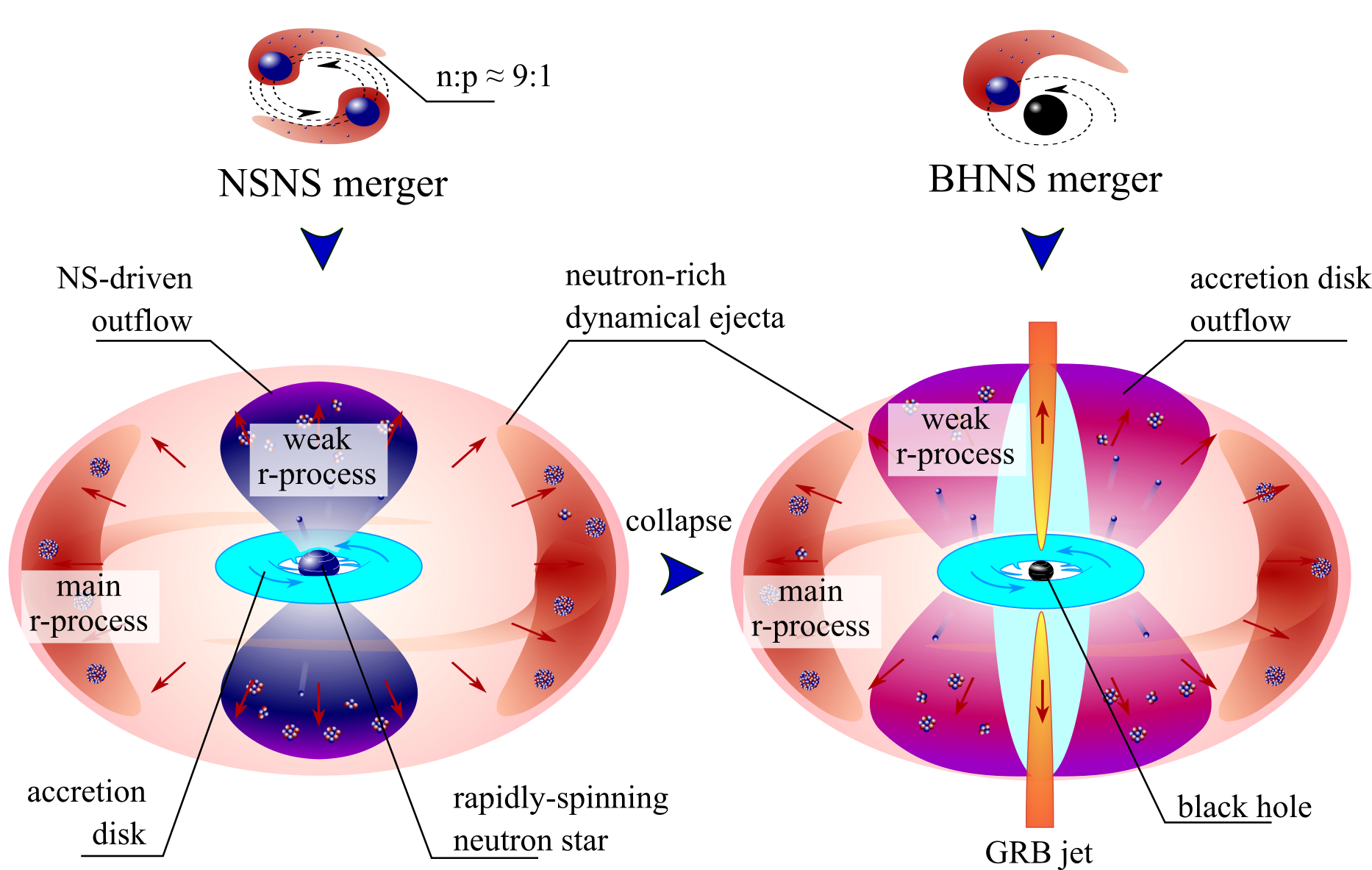A binary merger of neutron stars is an exciting possibility (some indirect evidence exists)

Another option is in the disk of a black hole neutron star binary

Lattimer & Schramm (1974) • Korobkin et al. (2012) • Figure from Mumpower et al. in prep. (2019)

Superheavy element production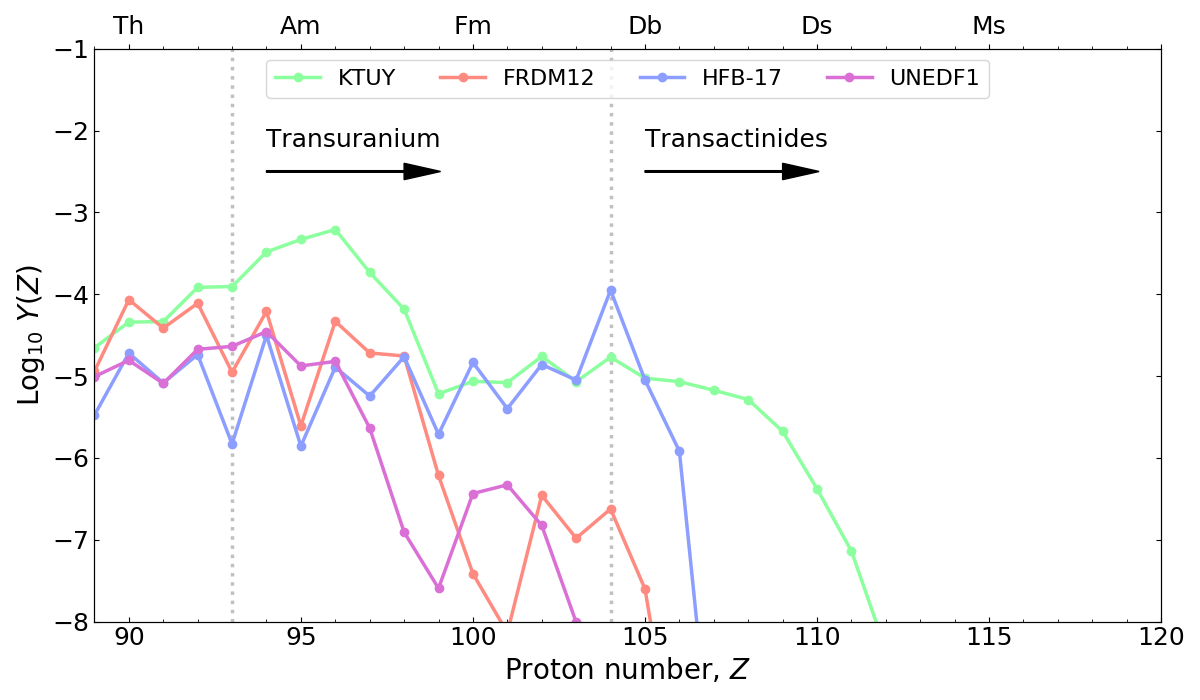Maximum heavy element production from tidal ejecta of a neutron star merger

A wide range of transuranic elements with some transactinide production is possible

Among other things, the details of SHE production depend on how the $r$-process terminates via nuclear fission

Extension of KTUY by H. Koura • Work in prep. (2019)

Nuclear fission for the $r$-process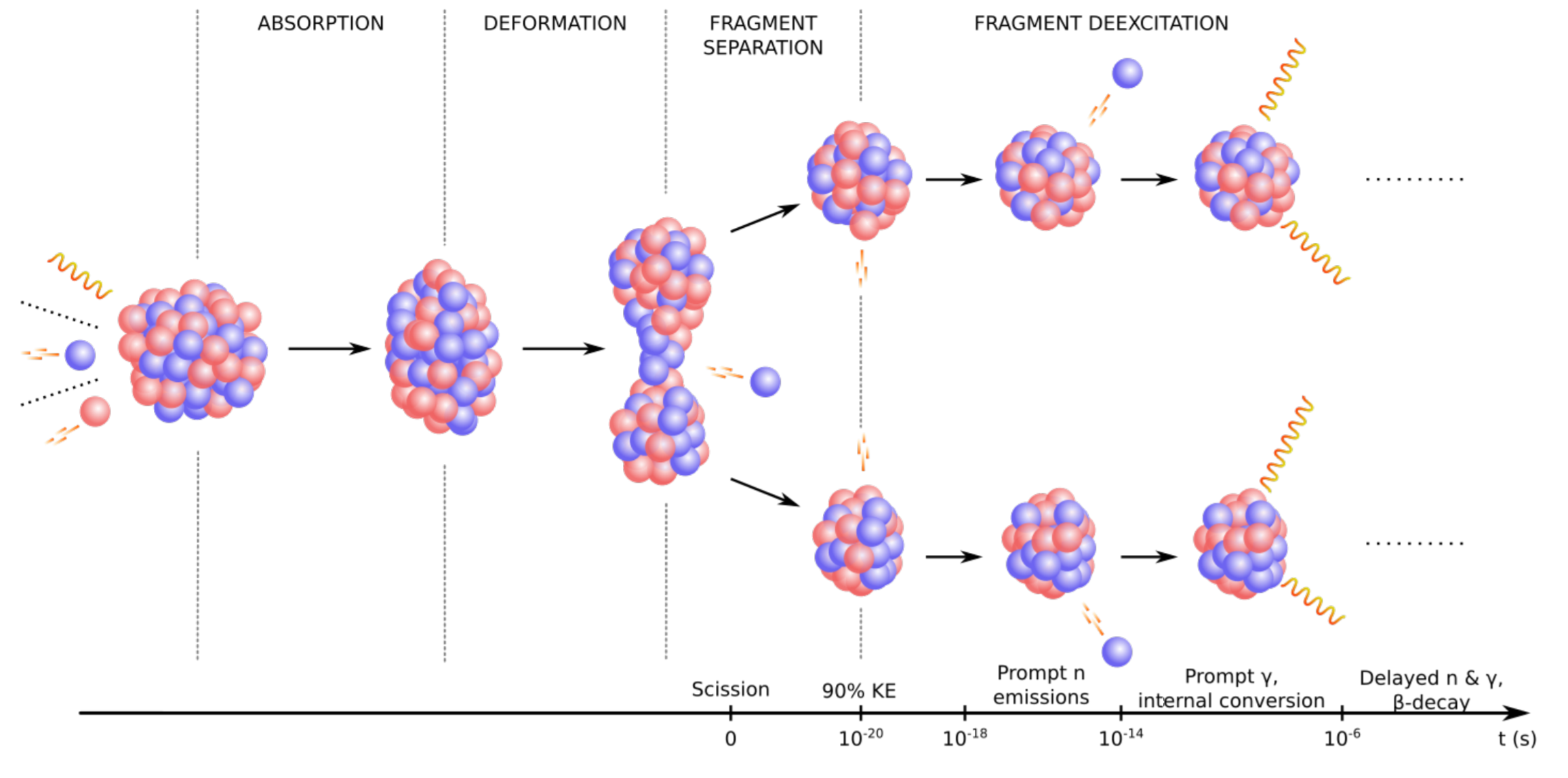Influence on the $r$-process:

Fission rates and branching determine re-cycling (robustness)

Fragment yields place material at lower mass number; barriers determine hot spots

Large Q-value ⇒ impacts thermalization and therefore possibly observations

Responsible for what is left in the heavy mass region when nucleosynthesis is complete ⇒ "smoking gun"

Holmbeck et al. ApJ 870 1 (2019) • Vassh et al. J. Phys. G (2019) • Figure from Verriere et al. in prep. (2019)

Fission Barrier Heights (FRLDM)(Maximum) FRLDM Barrier heights

Low barrier ↦ fission ⇑

$r$-process hot spots follow low barriers

Möller et al. PRC 91 024310 (2015)

Neutron-induced fissionUse statistical Hauser-Feshbach for competition between neutrons, $\gamma$s and fission

Barrier heights Möller (2015) / FRDM2012 masses

Large region that will fission cycle $r$-process

Mumpower & Kawano in prep. (2019)

$\beta$ -delayed fission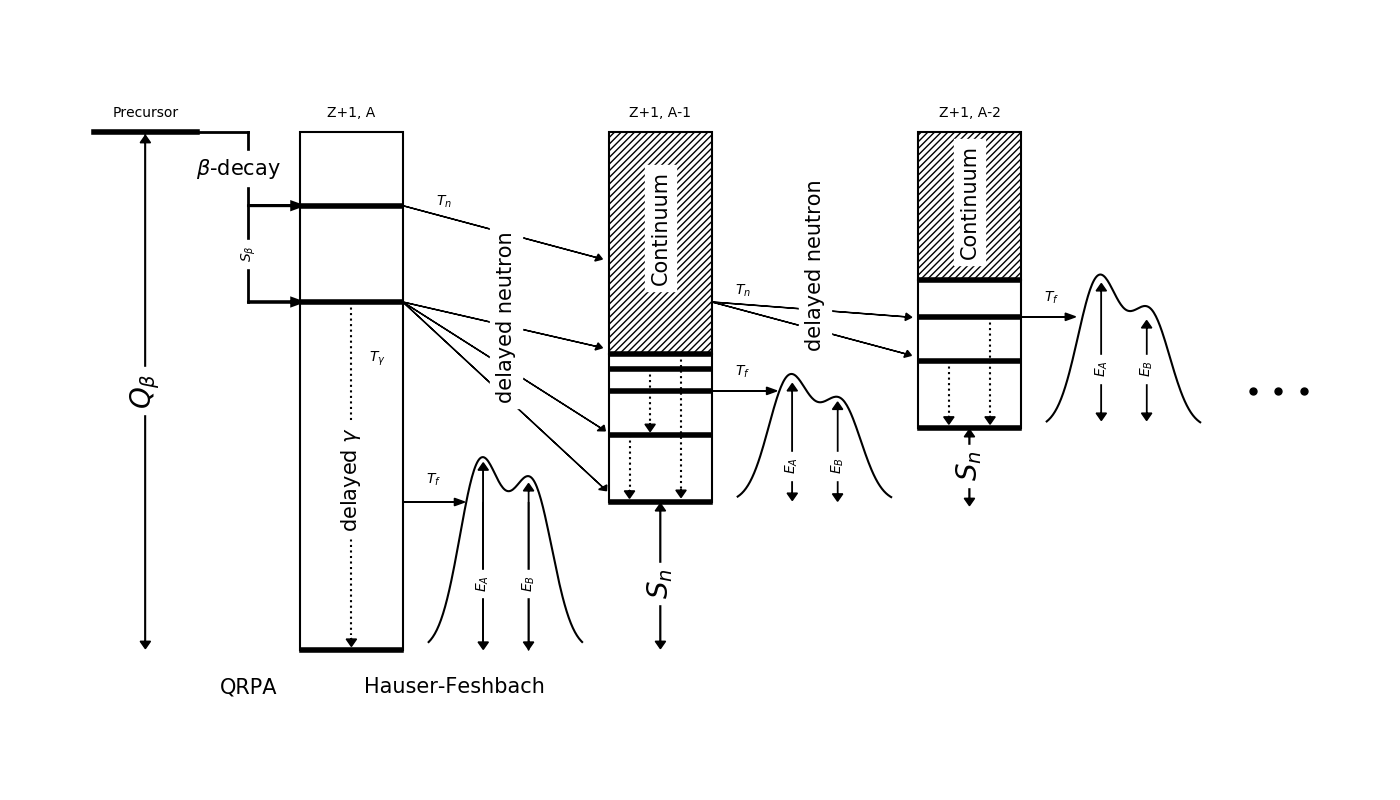We have recently extended our QRPA+HF model to describe $\beta$-delayed fission ($\beta$df)

Recall: Near the dripline $Q_{beta}$ ⇡ $S_{n}$ ⇣

Multi-chance $\beta$df: each daughter may fission

Kawano et al. PRC (2008) • Mumpower et al. PRC 94 064317 (2016) • Spyrou et al. PRL (2016)
Mumpower et al. ApJ (2018) • Möller et al. ADNDT 125 (2019) • Yokoyama et al. PRC (2019)

Cumulative $\beta$ df probability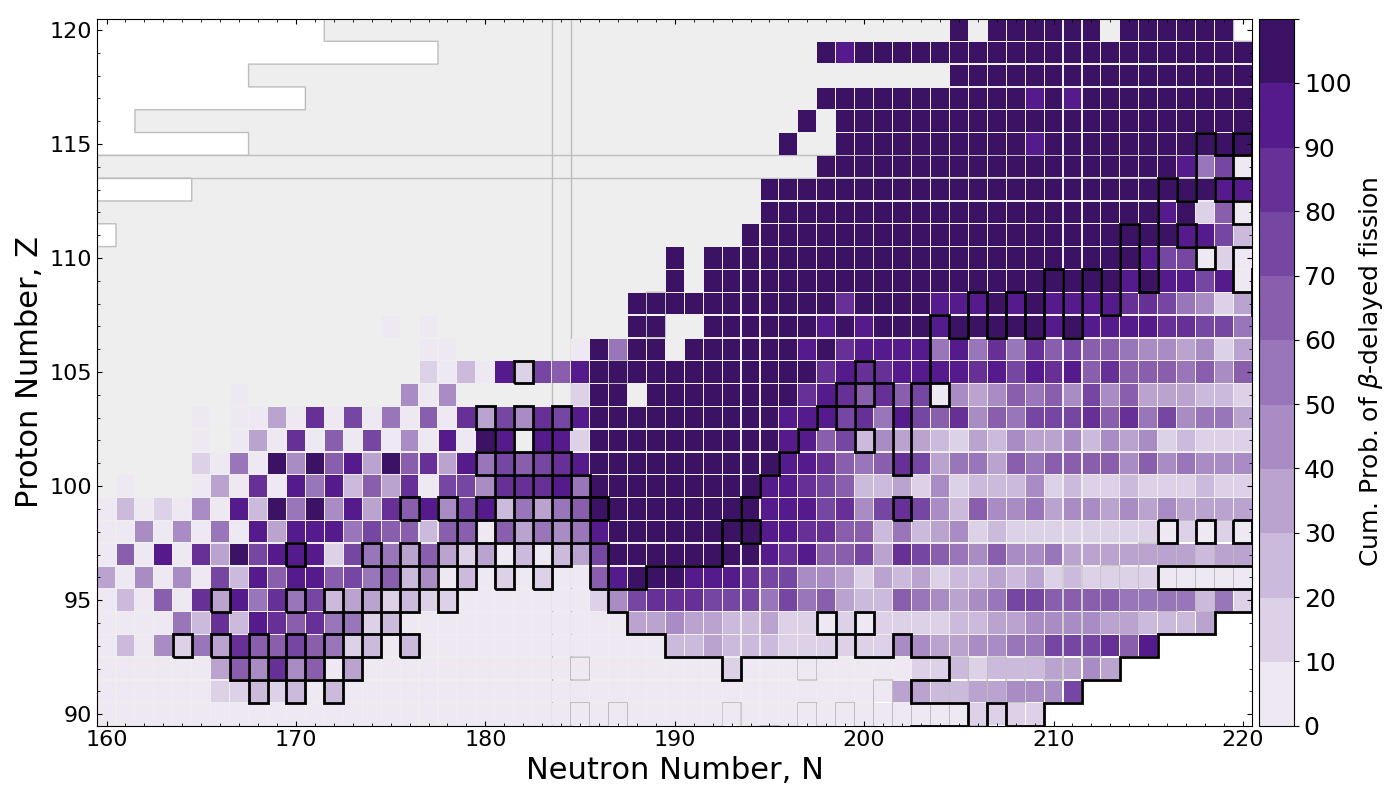$\beta$df occupies a large amount of real estate in the NZ-plane

Multi-chance $\beta$df outlined in black

Mumpower et al. ApJ 869 1 (2018)

Superheavies in the $r$-processNetwork calculation of tidal neutron star merger ejecta

$\beta$df alone may prevent the pathway from neutron-rich nuclei to the island of stability

Thielemann et al. Zeitschrift fur Physik A Atoms and Nuclei 309 4 (1983) • Mumpower et al. ApJ 869 1 (2018)

Fission yields across the chart of nuclides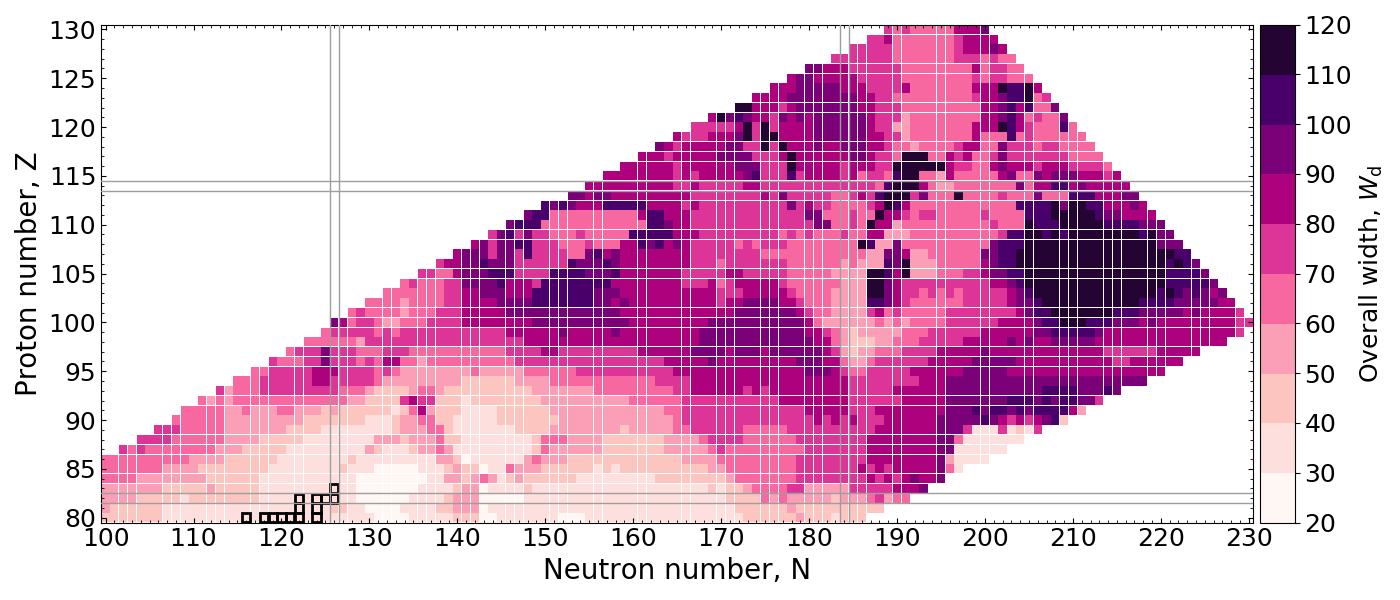We have calculated macro-micro fission yields across the chart of nuclides - including superheavies

Width of the mass distribution, $Y(A)$, shown above for each fissioning system - remarkable structure arises

Width of yield distributions increase with neutron-excess

Above results: Mumpower et al. arXiv:1911.06344 (2019) • GEF results: Vassh et al. J. Phys. G 46 065202 (2019)

Impact of new fission yields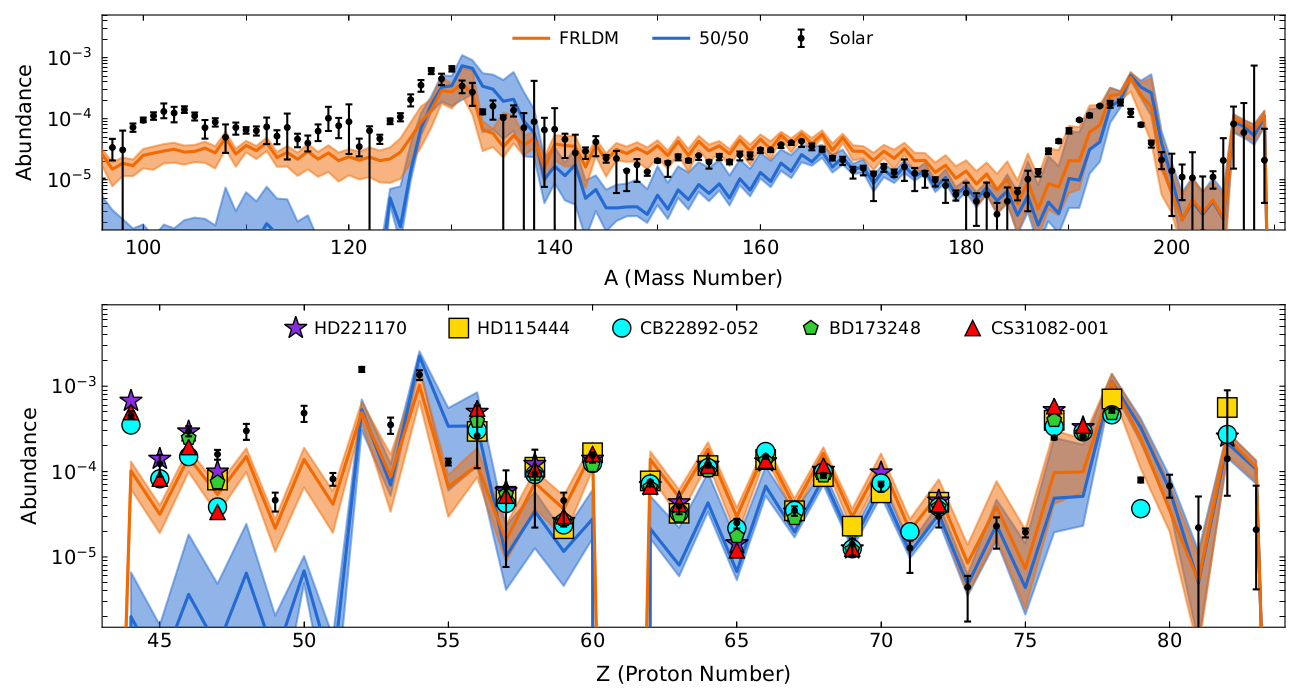Abundance output using common old nuclear fission data and our new model predictions

Co-production of light nuclei from $Z\sim 45$ to the actinides (dynamical merger ejecta only!)

Mumpower et al. arXiv:1911.06344 (2019) • Vassh et al. arXiv:1911.07766 (2019)

Is there a way to observe SHE in light curves?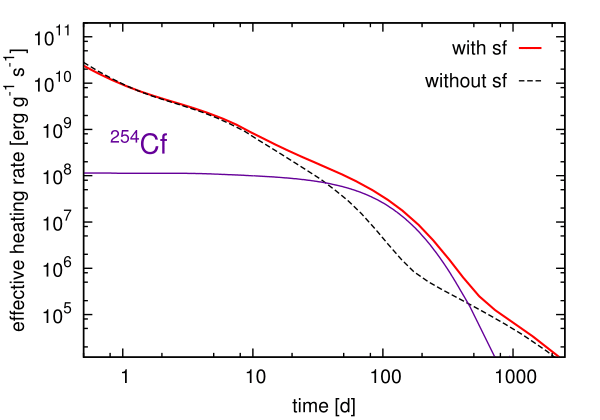Can we prove that very heavy elements nucleosynthesis has occurred in an event?... Maybe!

The spontaneous fission of $^{254}$Cf can be a primary contributor to nuclear heating at late-time epochs

The $T_{1/2}\sim 60$ days; found from nuclear weapons testing

Baade et al. PASP (1956) • Conway et al. JOSA (1962) • Wanajo et al. ApJL (2014) • Y. Zhu et al. ApJL 863 2 (2018)
Vassh et al. J. Phys. G (2019)

Production of $^{254}$Cf(Z=98)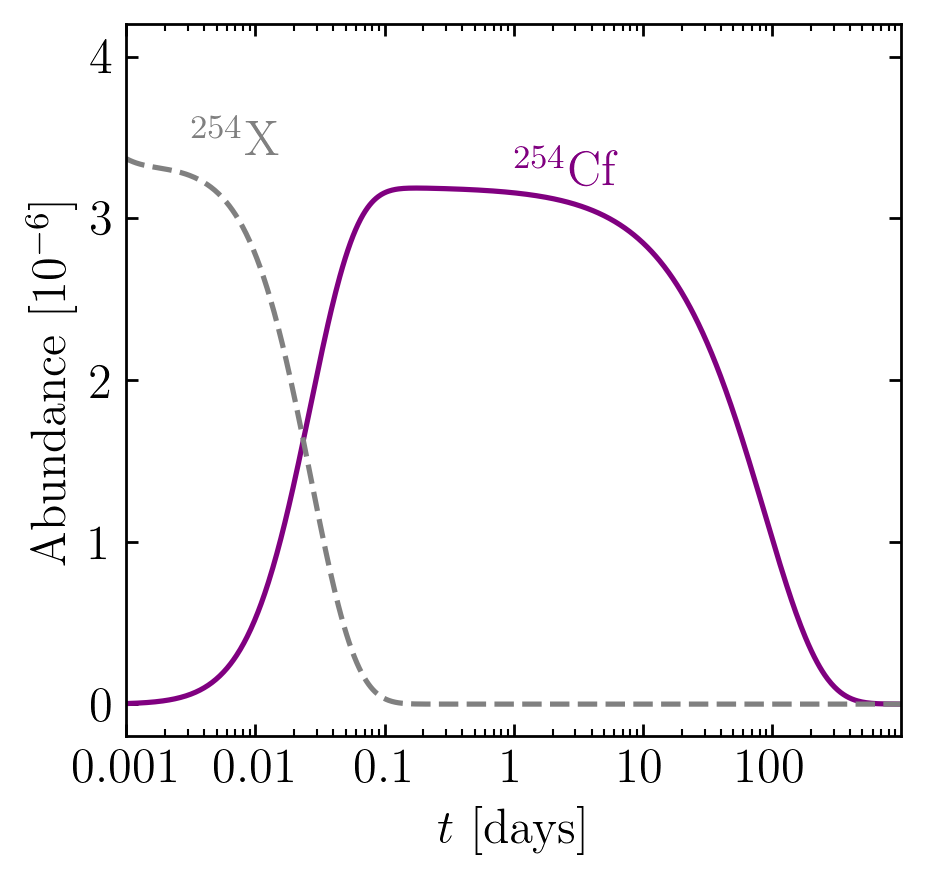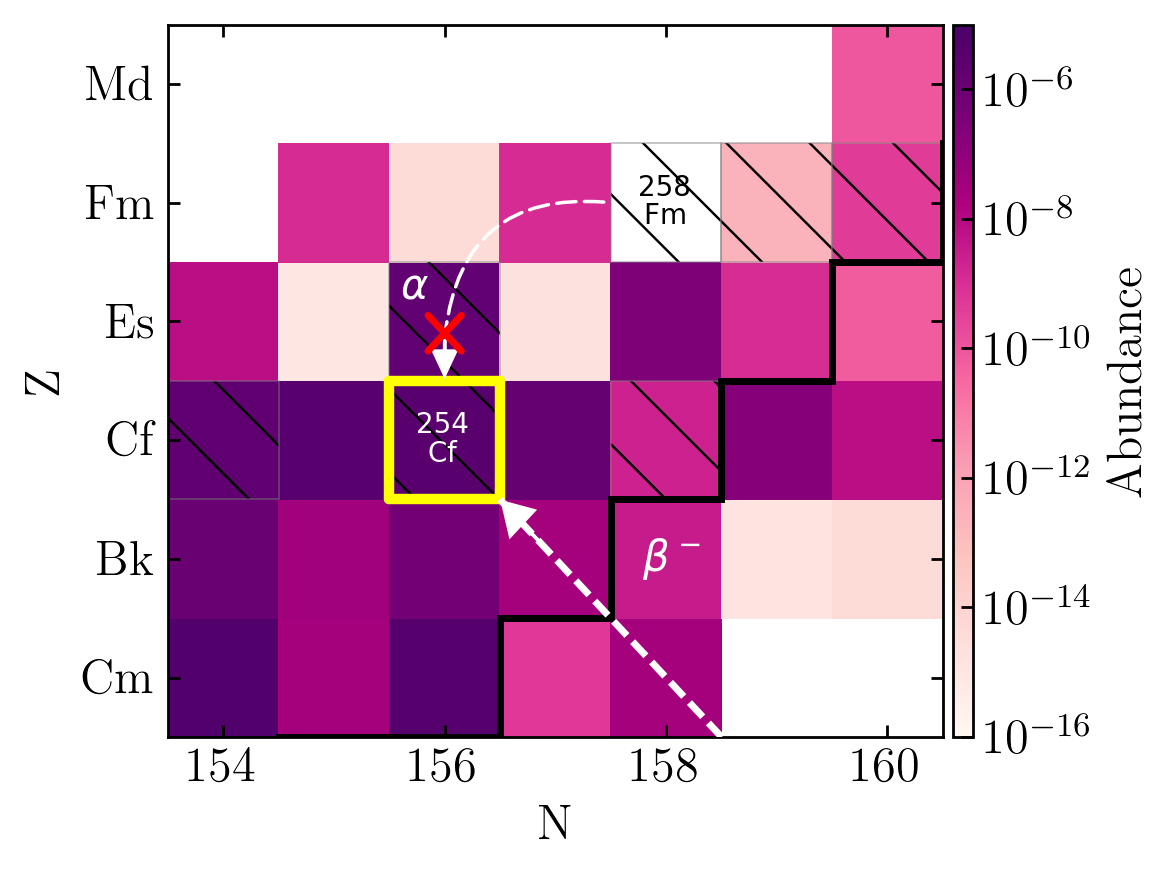Primary feeder seems to be from $\beta$-decay

Production of this nucleus been explored over a range of nuclear models; some high - some low

Remains to be seen if we can disentangle from other energy sources (e.g. puslar or accreetion fallback)

Wanajo et al. (2014) • Y. Zhu et al. ApJL 863 2 (2018) • Vassh et al. J. Phys. G (2019) • Wollaeger et al. ApJ (2019)

Observational Impact of Californium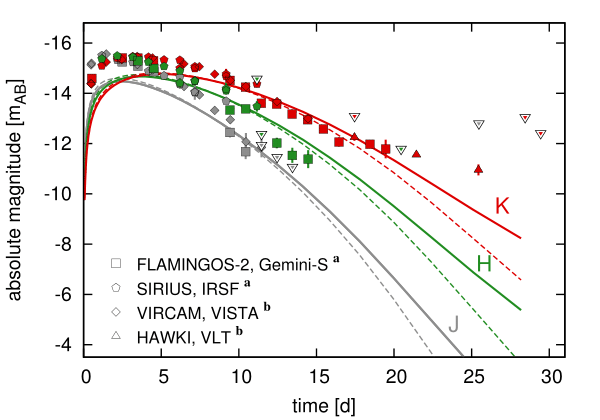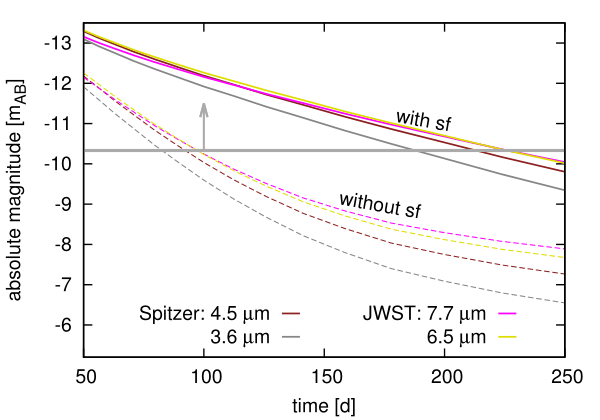Both near- and middle- IR are impacted by the presence of $^{254}$Cf

Late-time epoch brightness can be used as a proxy for heavy element nucleosynthesis

Future JWST will be detectable out to 250 days with the presence of $^{254}$Cf

This also has implications for merger morphology...

Wanajo et al. (2014) • Y. Zhu et al. ApJL 863 2 (2018) • Vassh et al. J. Phys. G (2019) • Wollaeger et al. ApJ (2019)

Special thanks to

My collaborators

J. Dolance, W. Even, C. Fontes, C. Fryer, E. Holmbeck, A. Hungerford, P. Jaffke, T. Kawano, O. Korobkin, J. Lippuner, G. C. McLaughlin, J. Miller, W. Misch, P. Möller, J. Randrup, T. Sprouse, R. Surman, N. Vassh, M. Verriere, R. Vogt, R. Wollaeger, Y. Zhu
& many more...

Student Postdoc CTA Staff FIRE PI

Summary

Production of superheavy elements in the $r$-process still remains an open question

The solution will require a coordinated, multi-disciplinary effort

Recent calculations show a wide range of transuranic and some transactinide production in low $Y_e$ ejecta

$\beta$-delayed fission may terminate pathways from neutron-rich nuclei to the island of stability

Influential, late-time nuclei such as $^{254}$Cf may provide insight into SHE production in nature

Currently: more theoretical effort, experiments, and observations are needed!

Results / Data / Papers @ MatthewMumpower.com